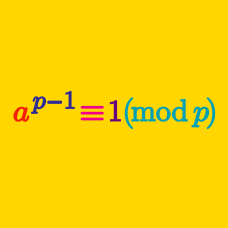Number Theory

# Modular Arithmetic - Problem Solving

What is the remainder of $\displaystyle {1776}^{2011!}$ upon division by $2000?$

What is the remainder of ${22}!$ upon division by $23?$

What is the remainder of $\displaystyle {144}^{10}$ upon division by $13?$

What is the remainder of $m$ satisfying $\frac{1}{1}+\frac{1}{2}+\frac{1}{3}+\cdots+\frac{1}{33}=\frac{m}{33!}$ upon division by $17 ?$

For three integers $x,$ $y$ and $z,$ which of the following can NOT be expressed as $x^2+y^2+5z^2 ?$

×